# Kinetic Temperature

The expression for gas pressure developed from kinetic theory relates pressure and volume to the average molecular kinetic energy. Comparison with the ideal gas law leads to an expression for temperature sometimes referred to as the kinetic temperature.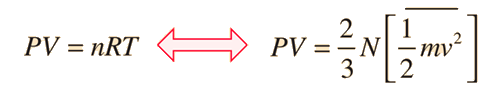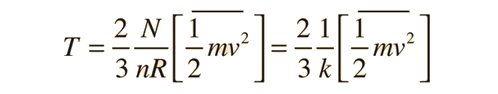where N is the number of molecules, n the number of moles, R the gas constant, and k the Boltzmann constant. The more familiar form expresses the average molecular kinetic energy:Show

It is important to note that the average kinetic energy used here is limited to the translational kinetic energy of the molecules. That is, they are treated as point masses and no account is made of internal degrees of freedom such as molecular rotation and vibration. This distinction becomes quite important when you deal with subjects like the specific heats of gases. When you try to assess specific heat, you must account for all the energy possessed by the molecules, and the temperature as ordinarily measured does not account for molecular rotation and vibration. The kinetic temperature is the variable needed for subjects like heat transfer, because it is the translational kinetic energy which leads to energy transfer from a hot area (larger kinetic temperature, higher molecular speeds) to a cold area (lower molecular speeds) in direct collisional transfer.

 Define constants Equipartition of energy Thermal energy
Index

Gas law concepts

Kinetic theory concepts

 HyperPhysics***** Heat and Thermodynamics R Nave
Go Back

# Molecular Speeds

From the expression for kinetic temperature### Calculation

substitution gives the root mean square (rms) molecular velocity: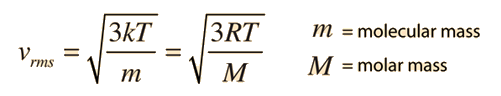From the Maxwell speed distribution this speed as well as the average and most probable speeds can be calculated.
Index

Kinetic theory concepts

 HyperPhysics***** Heat and Thermodynamics R Nave
Go Back

# Maxwell Speed Distribution

The speed distribution for the molecules of an ideal gas is given by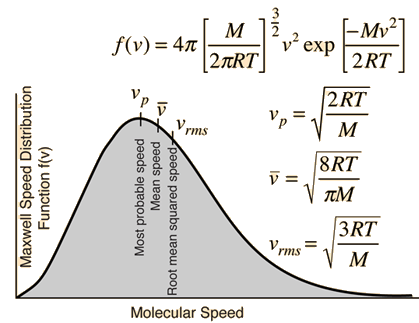From this function can be calculated several characteristic molecular speeds, plus such things as the fraction of the molecules with speeds over a certain value at a given temperature. It is used in calculating the rates of many phenomena.

### Calculation

Note that M is the molar mass and that the gas constant R is used in the expression. If the mass m of an individual molecule were used instead, the expression would be the same except that Boltzmann's constant k would be used instead of the molar gas constant R.

 Why does the probability peak at some finite value, when the average velocity is zero?
 Development of Maxwell speed distribution from Boltzmann distribution Some comments about developing the relationship
Index

Kinetic theory concepts

 HyperPhysics***** Heat and Thermodynamics R Nave
Go Back

# Molecular Speed Calculation

The speed distribution for the molecules of an ideal gas is given byThe calculation of molecular speed depends upon the molecular mass and the temperature. For mass m= amu M= kg/mol and temperature T=K T= C the three characteristic speeds may be calculated. The nominal average molecular mass for dry air is 29 amu.
Most probable speed = vp = m/s = km/hr = mi/hr
Mean speed=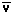=m/s = km/hr = mi/hr
RMS speed = vrms = m/s = km/hr = mi/hr
 Frequency of collisions Mean free path
Index

Kinetic theory concepts

 HyperPhysics***** Heat and Thermodynamics R Nave
Go Back

# Development of the Boltzmann Distribution

Statistical methods become a more precise way to study nature when the number of particles is large. So we expect that the description of the velocities of molecules in a gas will in fact be the most probable distribution, since we are dealing with particle numbers in the range of Avogadro's number. But this most probable distribution (the Maxwell-Boltzmann distribution) is subject to constraints, namely that the number of particles is constant and that the total energy is constant (conservation of energy). Maximizing the probability distribution subject to those constraints in general is a formidable mathematical task (see Richtmyer, et al., for example). One way to approach the solution in a more intuitive way is to deal with a physical example which we know - namely the physics of an atmosphere under the influence of gravity as reflected in the barometric formula. The following treatment follows the development by Rohlf.

In this approach we make use of the fact that the average kinetic energy of the molecules can be expressed in terms of the kinetic temperature. In addition, we know that conservation of energy in this case involves just the balancing of kinetic energy and gravitational potential energy so long as we treat the atmosphere as an ideal gas.

From the expression for kinetic temperaturewe have an experimentally tested expression for molecular kinetic energy. In the barometric formula: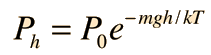we have a description of an ideal gas system which can be used to help develop a plausibility argument for the Maxwell velocity distribution. The steps in this process are as follows:

 Relate particle velocity to height. Relate particle flux to the velocity distribution. Relate velocity distribution to the barometric formula. Calculate the velocity distribution and normalize it.

For one direction in space this process yields the expression:and when all directions of velocity are included, it becomes the Maxwell speed distribution relationship:Why is this relationship skewed toward higher speeds while the one directly above is not?

It should be noted that although we used a gravity-dependent physical situation to obtain the velocity distribution, gravity does not appear in the final result. That is, the result obtained is a general one, not containing g. The barometric formula was simply used as a construct to relate the velocity distribution to energy and particle number constraints.

Index

Kinetic theory concepts

Reference
Rohlf
Sec 2-3

 HyperPhysics***** Heat and Thermodynamics R Nave
Go Back